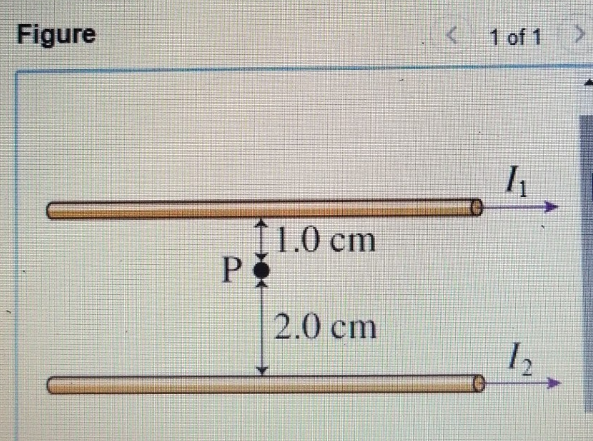# Problem: Consider the wires shown in (Figure 1). the upper wire carries the current I1 = 20A.What should the current I2 be in the lower wire such that the magnetic field at point P is zero?

###### FREE Expert Solution

Magnetic field around a wire carrying current:

$\overline{){\mathbf{B}}{\mathbf{=}}\frac{{\mathbf{\mu }}_{\mathbf{0}}\mathbf{i}}{\mathbf{2}\mathbf{\pi }\mathbf{r}}}$

At point P, the magnetic field is zero.

82% (403 ratings)###### Problem Details

Consider the wires shown in (Figure 1). the upper wire carries the current I1 = 20A.What should the current I2 be in the lower wire such that the magnetic field at point P is zero?